### INDUCTION

WALTER RITZ

#### Annales 242 (Oeuvres 397)

Let ds' be a linear element of one of the closed currents in which one can decompose the inductive current. Let V' be the speed of the element (which is at the same time that of the positive ions); we (Oeuvres 398) have calculated (27) the action of such an element on a mobile ion by supposing V'=0 and neglecting the accelerations. Let us go back to the calculations without these restrictions, but also ignoring the electrostatic action of the current, and, a fortiori, all the terms multiplied by the resultant charge E' of ds, which comes back to setting. Let F be the force exerted by the positive and negative charges of ds on a unit of charge placed in (xyz), of speed v. The electromotive force of induction produced in a conductor wire whose element is ds (dx,dy,dz) is determined by the total tangential component of F:In closed induced currents, we observe only the value of this integral taken along a closed contour. If therefore F contains terms of the form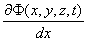, these terms will give a total differential, and consequently will disappear from the result.

To calculate F, let us start from formula (15). We can neglect the term independent of v,for all closed induced currents. The term containing w will be multiplied by E', and in consequence will obviously be nil; thus we will haveIn this expression, the terms independent of v will not figure in the operation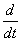, and their partial derivative in relation to x y z alone intervenes and gives, from that point, a nil result. There will remain(Oeuvres 399) The first term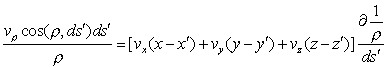gives, integrated by parts,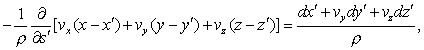so that we have in the end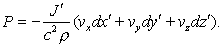In Lorentz’s theory, there exists a function L given by (20a) and which leads, by the same operation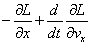to the expression of the force. The terms in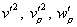of this expression again give zero

for a closed secondary circuit. The resulting value of L will thus be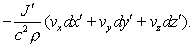Thus the two theories give, for two closed circuits in motion (for we have made no hypothesis on the movement of the induced circuit, and we have not supposed v parallel to ds), the same result, in accord with the experiment.

The more special hypotheses of Weber led, as we know, to the same results.

Magnets will be treated as in Lorentz’s theory.

One remark is nevertheless necessary. In order for Lorentz’s formula to conform with

experiment, it is necessary that only the tangential acceleration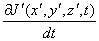play a role (in the case of variable currents), the normal acceleration, proportional to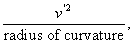being negligible beside it. Stated otherwise necessary thatmust be essentially reduced to its first term.

(Oeuvres 400) When the secondary circuit is open, there occur, as we know, potential differences; the study of several cases where these differences could be observed may easily be treated by the same methods used up to this point, and I won’t dwell on it.